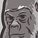# QlikView App Dev

Discussion Board for collaboration related to QlikView App Development.

Announcements
Join us for a live Q&A! September 21, 10 AM ET - Onboarding Fast in Qlik Sense SaaS! REGISTER
cancel
Showing results for
Did you mean:Creator III

## Percentage issue,

So i have a table bellow are the column and expression . Some how percentages total label is incorrect where as by category is correct

Column 1 Total

if(Sum({< Is={'T'}, [ CAT Name] = {'T I'} >} [ Sold] )>0,
Sum({< Is={'T'}>} [Sold] ))

Column 2  GRE

if(Sum({< Is={'T'}, [CAT Name] = {'T I'} >} [ Sold] )>0,
Sum({< Is={'T'}, [CAT Name] = {'T I'} >} [ Sold] ))

total percentages

if(Sum({< Is={'T'}, [CAT Name] = {'T I'} >} [ Sold] )>0,
Sum({< Is={'T'}, [CAT Name] = {'T I'} >} [Sold] ) / Sum({< Is={'T'} >} [Sold] ))

1 Solution

Accepted SolutionsMVP

May be this

```Sum({<Is={'T'}, [CAT Name] = {'T I'}>} [ Sold])
/
Sum(Aggr(
If(Sum({< Is={'T'}, [ CAT Name] = {'T I'} >} [ Sold]) > 0, Sum({<Is={'T'}>} [Sold]))
, \$(='[' & GetCurrentField([Group Name]) & ']'))))```
8 RepliesMVP

What is wrong? If 18.1% is not right then what is right?Creator III
Author

664518 of 2650572 is 24.95Creator III
Author

Opps i attached wrong  File . editing soonCreator III
Author

Please see the attachment now thanksMVP

Try this

```Sum({<Is={'T'}, [CAT Name] = {'T I'}>} [ Sold])
/
Sum(Aggr(  If(Sum({< Is={'T'}, [ CAT Name] = {'T I'} >} [ Sold]) > 0, Sum({<Is={'T'}>} [Sold])), Dimension))```Creator III
Author

Thanks Sunny, it  worked perfectly , however it has Cycle group  dimension , so it only works for one dimension . is there a way to works in all dimensionMVP

May be this

```Sum({<Is={'T'}, [CAT Name] = {'T I'}>} [ Sold])
/
Sum(Aggr(
If(Sum({< Is={'T'}, [ CAT Name] = {'T I'} >} [ Sold]) > 0, Sum({<Is={'T'}>} [Sold]))
, \$(='[' & GetCurrentField([Group Name]) & ']'))))```Creator III
Author

Thanks it worked out perfectly .Community Browser